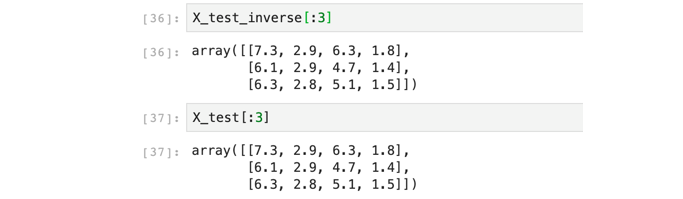DAY 4
0
AI & Data

# 咱們一起做資料清理和前處理

## 今日學習目標

• 資料如何清理
• 什麼是資料清理？
• 資料前處理的方式
• 為什麼資料要前處理呢？前處裡有何好處？
• 學習 Sklearn 中四種不同資料前處理方式
• StandardScaler (平均值和標準差)
• MinMaxScaler(最小最大值標準化)
• MaxAbsScaler（絕對值最大標準化）
• RobustScaler

## 載入相關套件

``````import pandas as pd
import numpy as np
import matplotlib.pyplot as plt
import seaborn as sns

np.set_printoptions(suppress=True)
``````

## 1) 載入資料集

``````iris = load_iris()
df_data = pd.DataFrame(data= np.c_[iris['data'], iris['target']],
columns= ['SepalLengthCm','SepalWidthCm','PetalLengthCm','PetalWidthCm','Species'])
df_data
``````

## 2) 檢查缺失值

``````X = df_data.drop(labels=['Species'],axis=1).values # 移除Species並取得剩下欄位資料
y = df_data['Species']
# checked missing data
print("checked missing data(NAN mount):",len(np.where(np.isnan(X))))
``````

``````checked missing data(NAN mount): 0
``````

## 3) 切割訓練集與測試集

``````from sklearn.model_selection import train_test_split

X_train, X_test, y_train, y_test = train_test_split(X, y, test_size=0.3, random_state=42, stratify=y)

print('train shape:', X_train.shape)
print('test shape:', X_test.shape)
``````

``````train shape: (105, 4)
test shape: (45, 4)
``````

## Standardization平均&變異數標準化

``````from sklearn.preprocessing import StandardScaler

scaler = StandardScaler()
X_train_scaled = scaler.fit_transform(X_train)
``````
``````# scaled之後的資料零均值，單位方差
print('資料集 X 的平均值 : ', X_train.mean(axis=0))
print('資料集 X 的標準差 : ', X_train.std(axis=0))

print('\nStandardScaler 縮放過後訓練集的平均值 : ', X_train_scaled.mean(axis=0))
print('StandardScaler 縮放過後訓練集的標準差 : ', X_train_scaled.std(axis=0))
``````

``````資料集 X 的平均值 :  [5.87333333 3.0552381  3.7847619  1.20571429]

StandardScaler 縮放過後訓練集的平均值 :  [ 0. -0. -0. -0.]
StandardScaler 縮放過後訓練集 X 的標準差 :  [1. 1. 1. 1.]
``````

``````X_test_scaled = scaler.transform(X_test)

print('\nStandardScaler 縮放過後測試集的平均值 : ', X_test_scaled.mean(axis=0))
print('StandardScaler 縮放過後測試集的標準差 : ', X_test_scaled.std(axis=0))
``````

``````StandardScaler 縮放過後測試集的平均值 :  [0.40925926 0.44259259 0.44750958 0.45185185]
StandardScaler 縮放過後測試集的標準差 :  [0.20457725 0.15915694 0.29647499 0.30224923]
``````

``````# 將縮放的資料還原
X_test_inverse = scaler.inverse_transform(X_test_scaled)
``````## MinMaxScaler最小最大值標準化

``````from sklearn.preprocessing import MinMaxScaler

scaler = MinMaxScaler()
X_train_scaled = scaler.fit_transform(X_train)
``````
``````# scaled 之後的資料最小值、最大值
print('資料集 X 的最小值 : ', X_train.min(axis=0))
print('資料集 X 的最大值 : ', X_train.max(axis=0))

print('\nStandardScaler 縮放過後訓練集的最小值 : ', X_train_scaled.min(axis=0))
print('StandardScaler 縮放過後訓練集的最大值 : ', X_train_scaled.max(axis=0))
``````

``````資料集 X 的最小值 :  [4.3 2.  1.1 0.1]

StandardScaler 縮放過後訓練集的最小值 :  [0. 0. 0. 0.]
StandardScaler 縮放過後訓練集的最大值 :  [1. 1. 1. 1.]
``````
``````X_test_scaled = scaler.transform(X_test)

print('\nStandardScaler 縮放過後測試集的最小值 : ', X_test_scaled.min(axis=0))
print('StandardScaler 縮放過後測試集的最大值 : ', X_test_scaled.max(axis=0))
``````
``````StandardScaler 縮放過後測試集的最小值 :  [ 0.02777778  0.125      -0.01724138  0.04166667]
StandardScaler 縮放過後測試集的最大值 :  [0.83333333 0.83333333 0.89655172 0.95833333]
``````

## MaxAbsScaler絕對值最大標準化

MaxAbsScaler 與 MinMaxScaler 類似，所有數據都會除以該列絕對值後的最大值。 數據會縮放到到[-1,1]之間。

``````from sklearn.preprocessing import MaxAbsScaler

scaler = MaxAbsScaler().fit(X)
X_scaled = scaler.transform(X)
``````
``````X_test_scaled = scaler.transform(X_test)
``````

## RobustScaler

``````from sklearn.preprocessing import RobustScaler

scaler = RobustScaler().fit(X)
X_scaled = scaler.transform(X)
``````
``````X_test_scaled = scaler.transform(X_test)
``````